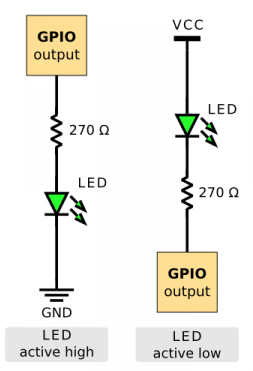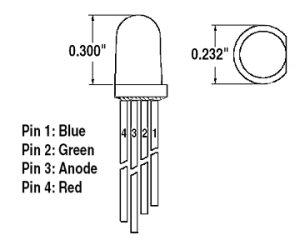##### Page tree
Go to start of banner

# LEDs

Light Emitting Diodes# Typical Voltage Drops

ColorVoltage Drop
Red1.7
Bright Red1.9
Orange2.0
Green2.1
Bright White3.4
Blue3.4

# Using LEDs

By syncing the LED to the GPIO, you can remove the constraint of the GPIO max current.# Multi-Color LEDs# Calculating Resistance Needed

For brightest LED without exceeding max GPIO current of 12 mA (ESP8266):

V = I * R

R = V / I = 3.3/0.012 = 275 ohms

Question:

Should we consider the voltage drop in the following calculation? Should it be:

V = I * R

R = ( Vss - Vdrop ) / I = (3.3 - 1.7) / 0.012 = 133 ohms

• No labels